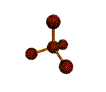##Mr. Rapp's Chemistry Help Site
 Grades  Graphing Tips  Online 3-D Laboratory  Reference Desk  AP Chemistry Test  News from Science  Links  After School Help  Fun Stuff  Teachers' Lounge    BHSS Chemistry  E-mail Mr. RappStudy Guide:  Solutions "If you're not a part of the solution, then you're a part of the precipitate." --Steven Wright   Students should be able to... Define:solute, solvent, solution, electrolyte, nonelectrolyte, types of solutions (combination of phases), saturated, unsaturated, supersaturated, dilute, concentrated, aqueous, etc. List factors that affect solubility Calculate Molarity. Calculate molality. Calculate % by mass Calculate changes in freezing point and boiling point in molecular solutes Calculate changes in freezing point and boiling point in ionic solutes Make solutions of known volume and Molarity (laboratory) Standardize a NaOH solution by titration (laboratory) Two sections: · Part I: Fill in the blank - terms; definition will be given, you provide the term name · Part II: Problem Solving Sample problems! 1. A solution is prepared by mixing 1.0 grams of benzene (C6H6) in 100 g of water to create a solution total volume of 100 ml. Calculate the molarity, mass percent, and molality of benzene in the solution. (0.128M, 0.99%, 0.128m) 2. 10 grams of salt (NaCl) is added to 100 mL of water. What are the new freezing and boiling points? (Kb = .51 oC kg/mol, Kf = 1.86 oC kg/mol) (-6.3 deg C, 102 deg C) 3. Calculate the molarity of a solution that contains 1.22 grams of hemoglobin (MW = 68300) in 165 ml of solution. (1.08 X 10-4 M) 4. How much solute was used to make a 40% solution of NaCl in H2O if 150 grams of water were used? (100. g NaCl) 5. Find the molality of a solution of 85 g KNO3 and 125 g H2O. (6.73 m) 6. What is the freezing point of a solution containing 202 g C12H22O11 and 750 g of H2O? (-1.46 deg C) 7. What is the boiling point of a solution containing 110 g CaSO4 and 234 g H2O? (103.59 deg C) 8. How many grams of Al(OH)3 are needed to make 300 mL of a 0.43M solution? (10.1 g) 9. How many moles of PbSO4 are needed to make 200 mL of a 3.7M solution? (0.74 mol) 10. What is the volume, in mL, of a 1.7M solution that contains water and 284 grams of C6H12O6? (930 mL)E-mail Mr. Rapp | BHSS Chemistry Web SiteLast updated on April 28, 2006 9:18 AM